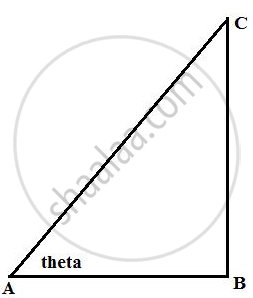# In Right-angled Triangle Abc; B = 90°. Find the Magnitude of Angle A, If: Bc is Sqrt(3) Times of Ab. - Mathematics

Sum

In right-angled triangle ABC; ∠ B = 90°. Find the magnitude of angle A, if:
BC is sqrt(3) times of AB.

#### Solution

Consider the figureAgain from the figure

"BC"/"AB" = sqrt(3)

tan θ = sqrt(3)

tan θ = tan 60°

θ = 60°

Therefore, magnitude of angle A is 30°

Concept: Solution of Right Triangles
Is there an error in this question or solution?

#### APPEARS IN

Selina Concise Mathematics Class 9 ICSE
Chapter 24 Solution of Right Triangles [Simple 2-D Problems Involving One Right-angled Triangle]
Exercise 24 | Q 14.2 | Page 304